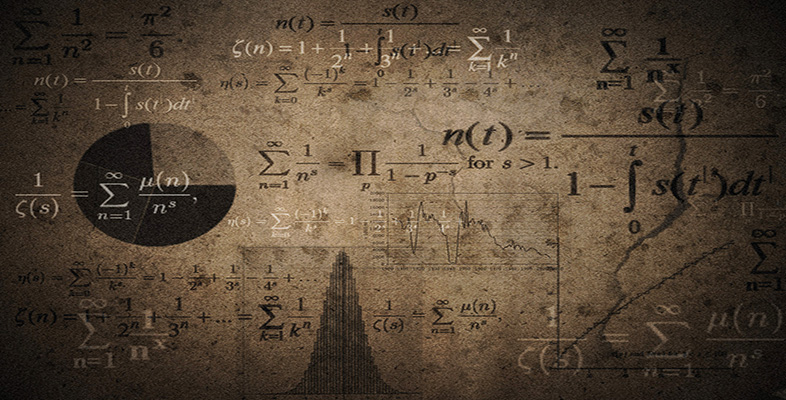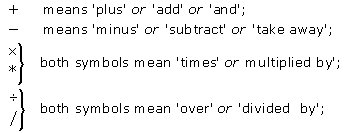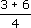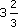Language, notation and formulas

This free course is available to start right now. Review the full course description and key learning outcomes and create an account and enrol if you want a free statement of participation.

Free course

# 2.6.1 Arithmetical symbols

You will have already met the symbols for the basic arithmetical operations, which are +, −, × and ÷, but you may not have met some of the alternative ways of writing × and ÷.

To recap, the main symbols for arithmetical operations are:There are other alternatives to × and * for multiplication. Sometimes, provided there is no risk of ambiguity, no symbol is used, just as sometimes the words ‘multiplied by’ or ‘times’ can be omitted in speech: for example, two fours or five fives means two times four or five times five, respectively. So, instead of writing 3 × (4 + 5), you can just write 3(4 + 5) and save one symbol. Mathematicians like to be as concise as possible! Brackets avoid ambiguity in mathematical expressions.

An alternative to ÷ and / for division is a fractional notation: for example,means ‘add three and six, and then divide by four’. The slash symbol / is sometimes used for fractions: thus, three-quarters may be written as 3/4. Many calculators use this notation to display fractions.

## Example 4

What do the following expressions mean?

• (a) 5/(3 + 2)

• (b) 5/3 + 2

• (c) 4(8 − 5)

• (a) Divide 5 by the sum of 3 and 2. (This gives 1.)

• (b) Divide 5 by 3 and then add to 2, oradded to 2. (This gives.)

• (c) Multiply 4 by the difference between 8 and 5. (This gives 12.)

MU120_4M6